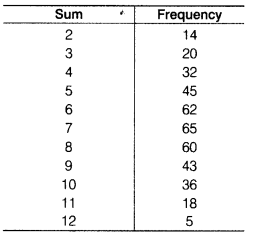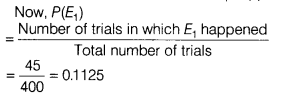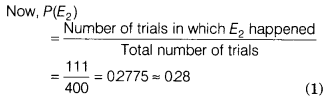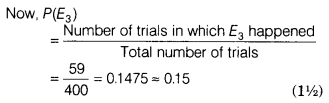# Two dice are thrown simultaneously 400 times. Each time, the sum of two numbers

Two dice are thrown simultaneously 400 times. Each time, the sum of two numbers appearing on their tops is noted and recorded as given in the following tableIf the dice are thrown once more, then what is the probability of getting a sum
(i) 5?
(ii) less than or equal to 5?
(iii) more than 9?

Given, two dice are thrown simultaneously 400 times.
∴ Total number of trials = 400
(i) Let E1 be the event of getting a sum 5.
Then, number of trials in which E1 happened = 45(ii) Let E2 be the event of getting a sum less than or equal to 5.
Then, number of trials in which E2 happened = 14 + 20 + 32 + 45 = 111(iii) Let E3 be the event of getting a sum more than 9.
Then, number of trials in which E3 happened = 36+18+5=59